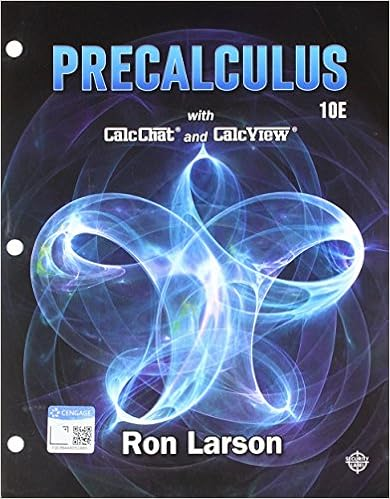# When a ump or umpu test exists an lr test is often

• Notes
• 78
• 100% (2) 2 out of 2 people found this document helpful

This preview shows page 37 - 39 out of 78 pages.

##### We have textbook solutions for you!
The document you are viewing contains questions related to this textbook.The document you are viewing contains questions related to this textbook.
Chapter 9 / Exercise 45
Precalculus
Hostetler/LarsonExpert Verified
When a UMP or UMPU test exists, an LR test is often the same as thisoptimal test. For a real-valuedθ, we have the following result.Proposition 6.5.Suppose thatXhas the p.d.f. given by (6.10) w.r.t. aσ-finite measureν, whereηis a strictly increasing and differentaible functionofθ.(i) For testingH0:θθ0versusH1:θ>θ0, there is an LR test whoserejection region is the same as that of the UMP testTgiven by (6.11).(ii) For testing the hypotheses in (6.12), there is an LR test whose rejectionregion is the same as that of the UMP testTgiven by (6.15).(iii) For testing the hypotheses in (6.13) or (6.14), there is an LR testwhose rejection region is equivalent toY(X)<c1orY(X)>c2for someconstantsc1andc2.Proof.(i) Letˆθbe the MLE ofθ. Note that(θ) is increasing whenθˆθand decreasing whenθ>ˆθ. Thus,λ(X) =braceleftBigg1ˆθθ0(θ0)(ˆθ)ˆθ>θ0.Thenλ(X)< cis the same asˆθ > θ0and(θ0)/ℓ(ˆθ)< c.From thediscussion in§4.4.2,ˆθis a strictly increasing function ofY.It can beshown that log(ˆθ)log(θ0) is strictly increasing inYwhenˆθ>θ0andstrictly decreasing inYwhenˆθ < θ0(exercise).Hence, for anyd∈ R,ˆθ>θ0and(θ0)/ℓ(ˆθ)<cis equivalent toY >dfor somec(0,1).(ii) The proof is similar to that in (i). Note thatλ(X) =braceleftBigg1ˆθ<θ1orˆθ>θ2max{(θ1),ℓ(θ2)}(ˆθ)θ1ˆθθ2.
##### We have textbook solutions for you!
The document you are viewing contains questions related to this textbook.The document you are viewing contains questions related to this textbook.
Chapter 9 / Exercise 45
Precalculus
Hostetler/LarsonExpert Verified
4306. Hypothesis TestsHenceλ(X)<cis equivalent toc1<Y <c2.(iii) The proof for (iii) is left as an exercise.Proposition 6.5 can be applied to problems concerning one-parameterexponential families such as the binomial, Poisson, negative binomial, andnormal (with one parameter known) families. The following example showsthat the same result holds in a situation where Proposition 6.5 is not ap-plicable.Example 6.20.Consider the testing problemH0:θ=θ0versusH1:θnegationslash=θ0based on i.i.d.X1,...,Xnfrom the uniform distributionU(0). We nowshow that the UMP test with rejection regionX(n)0orX(n)θ0α1/ngiven in Exercise 19(c) is an LR test.Note that(θ) =θnI(X(n),)(θ).Henceλ(X) =braceleftbigg(X(n)0)nX(n)θ00X(n)0andλ(X)<cis equivalent toX(n)0orX(n)0<c1/n. Takingc=αensures that the LR test has sizeα.More examples of this kind can be found in§6.6.The next exampleconsiders multivariateθ.Example 6.21.Consider normal linear model (6.38) and the hypothesesin (6.49). The likelihood function in this problem is(θ) =(12πσ2)n/2expbraceleftbig12σ2bardblXbardbl2bracerightbig,whereθ= (β,σ2). Letˆβbe the LSE defined by (3.27). SincebardblXbardbl2bardblXZˆβbardbl2for anyβ,(θ)(12πσ2)n/2expbraceleftBig12σ2bardblXZˆβbardbl2bracerightBig.
•••